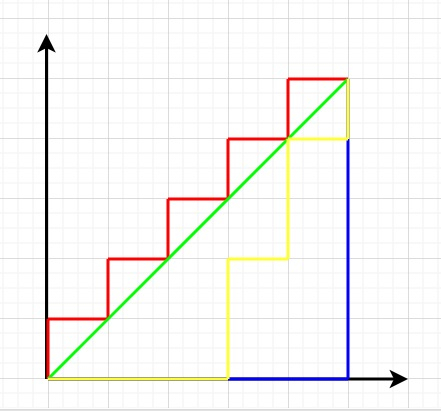# Count paths with distance equal to Manhattan distance in C++

We are given variables x1, x2, y1, y2 representing two points on a 2D coordinate system as (x1, y1) and (x2, y2). The goal is to find all the paths that will have distance equal to the Manhattan distance between these two points.

## Manhattan Distance

Manhattan Distance between two points (x1, y1) and (x2, y2) is −

MD= |x1 – x2| + |y1 – y2|

Let’s take A= |x1 – x2| and B= |y1 – y2|

All paths with Manhattan distance equal to MD will have edges count as (A+B). A horizontal edge and B vertical edges. So possible combination of (A+B) edges divided into 2 groups will be ( A + B )CB = ( A+B )! / (A!)(B!)Let us understand with examples

Input

Output − Count of possible moves in the given direction in a grid are − 6

Explanation

Choosing move {1,1} = (1,1) → (2,2) → (3,3) - 3 steps
Choosing move {0,-1} = (3,2) → (3,3) → (3,2) → (3,1) - 3 steps
Total 6 steps.

Input − A = 4, B = 4, x =2, y = 2; moves = {2, 1}, { -2, -3 }

Output − Count of possible moves in the given direction in a grid are − 2

Explanation

Choosing move {2,1} = (2,2) → (4,3) - 2 steps
Choosing move {-2,-3} = (2,0) X out of bound
Total 2 steps.

## The approach used in the below program is as follows

In this approach, we will create a vector representing steps as pair<int,int>. Start traversing from point x,y. Choose a step from the vector and choose the minimum of the value taken in both directions (x axis and y axis). The minimum chosen will allow more moves to take.

To move in a particular direction, if the cur position x ( or y ) is > n (or m) then the number of moves to reach n (or m) is ( n - cur position )/x. If it is less then the number of moves to reach 1 is ( cur position - 1 )/x.

• Take an array arr[] containing 0’s and 1’s.

• Function count_cars(int arr[], int size) takes the array and length as input and returns the count of passing cars.

• Take the initial count as 0.

• Traverse array from index i=0 to i<length-1.

• If arr[i] is 0, traverse array again from index j=i+1 to j<length.

• For each arr[j] as 1 increment count as pair (arr[i],arr[j]) is (0,1) and i<j.

• At last we will get the total count.

• Return count as result.

## Example

Live Demo

#include <bits/stdc++.h>
using namespace std;
long long int bio_coeff(int A, int B){
long long int temp = 1;
if (B > A - B){
B = A - B;
}
for (int i = 0; i < B; ++i){
temp = temp * (A - i);
temp = temp / (i + 1);
}
return temp;
}
long long int Manhattan_distance(int x1, int y1, int x2, int y2){
int A = abs(x1 - x2);
int B = abs(y1 - y2);
int count = bio_coeff(A + B, B);
return count;
}
int main(){
int x1 = 6, y1 = 8, x2 = 2, y2 = 10;
cout<<"Count of paths with distance equal to Manhattan distance are: "<<
Manhattan_distance(x1, y1, x2, y2);
return 0;
}

## Output

If we run the above code it will generate the following output −

Count of paths with distance equal to Manhattan distance are: 15

Updated on: 02-Dec-2020

268 Views